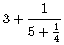# Countability of Rational Numbers via Continued Fractions

Every positive rational number can be written as a finite (simple) continued fraction. On their part, continued fractions could be written in at least two finite alphabets, say,

{0, 1, 2, 3, 4, 5, 6, 7, 8, 9, +, -}

where "-" denotes the bar, like inor in

{0, 1, 2, 3, 4, 5, 6, 7, 8, 9, [, ], ;, ,}

which includes a comma and a semicolon, like in the shorthand notations

[3; 5, 4].

The Countability Principle then assures that the number of positive rational numbers is countable.

Jerzy Czyz and William Self came up with a less direct way of counting the rationals with the help of continued fraction, but theirs has the advantage of being constructive.

Czyz and Self define their mapping in three steps.

First, a natural number is written in binary, with the first digit (which is always 1) removed. This maps natural numbers onto the set of finite binary strings. The mapping is 1-1.

Next, a binary string of length, say k, is expanded by k + 1 dots in front, at the end, and in between the digits. For example, 001000101 will appears as ·0·0·1·0·0·0·1·0·1·. (The empty string will be replaced with a single dot.)

This is an auxiliary representation of an integer from which the authors remove all zeros so that 001000101 will be written as ···1····1··1·, which is reminiscent of a method of partitioning a number (k + 1 in this case) into the sum of smaller numbers. Here we count the sequence of the addends, {3, 4, 2, 1}.

The second step is almost over but two amends need to be taken. First, the way the partition was formed, the first number in the resulting sequence is never 0 but we need to allow that. We thus subtract 1 from the first number in the sequence.

The second modification is necessary because representation of a rational number as a continued fraction is not unique. For example, the last 1/4 at the beginning of the page could be split into 1/(3 + 1/1). To preclude the ambiguity, we add 1 to the last number in the sequence. (This is of course done only if there are actual fractions, i.e., when the number of terms in the sequence is greater than 1.)

To finalize, 001000101 is mapped onto {2, 4, 2, 2}.

Finally, the sequence of integers from the previous step is interpreted as a continued fraction such that, e.g., {2, 4, 2, 2} is mapped onto

[2; 4, 2, 2] = 2 + 1/(4 + 1/(2 + 1/2)) = 49/22.

(This is why we wanted to allow for the first digit to be zero.)

Now, note that the binary string 001000101 I used in the examples corresponds to the binary integer 1001000101 which is 581 in the decimal system which is assigned to the fraction 49/22.

The first sixteen rational numbers in this enumeration are### References

1. J. Czyz and W. Self, The Rationals Are Countable: Euclid's Proof, The College Mathematics Journal, Vol. 34, No. 5 (Nov., 2003), pp. 367-369### Countability of Rational Numbers